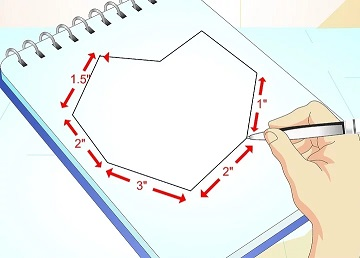### C - Can you draw it?

##### Time & Memory limits:(details)

Determine if an $N$-sided closed polygon (not necessarily convex) with positive area (i.e., non degenerate) and sides of length $L_1$, $L_2$,...,$L_N$ can be drawn in a two-dimensional plane.#### Input

The first line of the input contains the integer $N$ ($1 \leq N \leq 10$). The second line contains $N$ space-separated integers $1 \leq L_i \leq 100$.

#### Output

If an $N$-sided polygon satisfying the condition can be drawn, print Yes; otherwise, print No.

#### Sample test(s)

Input
4 3 8 5 1
Output
Yes
Input
4 3 8 4 1
Output
No
Input
10 1 8 10 5 8 12 34 100 11 3
Output
No